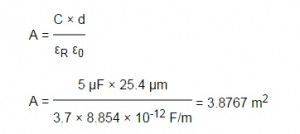Problem 1—When designing a pair of band-splitting filters (for, say, an audio crossover), why is it important to match frequencies of the –3-dB points of the low-pass and high-pass responses?

Answer 1—The cutoff frequencies of the two filters should be the same so that the overall frequency response when the filter outputs are recombined is flat and has no phase shift. For example, if you feed the cutoff frequency into both filters and then combine the results again, the output will be the same level as the input (0-dB overall gain). As long as the “order” of the two filters is the same (they have the same roll-off slope), the gain will be flat across the entire transition band of frequencies.

What’s really going on is this: A filter’s –3-dB point is where the output has half the power of the input signal, which means that the output voltage is 1/sqrt(2) times the input voltage. The –3-dB point is also where the output signal is phase shifted by 45°. It lags by 45° in the low-pass filter and leads by 45° in the high-pass filter. This means that the outputs of the two filters have a total phase shift of 90° relative to each other.

When you add two sinewaves that have the same amplitude and a 90° phase shift, you don’t get double the voltage. You get sqrt{2} times the voltage. You also get a waveform that has a phase midway between the two signals being added.

So, the final amplitude is sqrt{2}/sqrt{2} times the original input voltage, and the final phase is midway between 45° and –45°, or 0°. In other words, you get the original sinewave back exactly.

Problem 2—A certain portable stereo unit runs for about 12 h on a set of LR20 (D-size alkaline) batteries. If you want to extend the stereo’s run-time, is it better to simply use multiple sets of batteries sequentially, or to connect them all in series-parallel to create one big battery pack?

Answer 2—In general, batteries provide greater capacity at lower average currents. This is partly due to the battery’s internal chemistry, but largely due to the simple fact that less power is wasted in the internal resistance of the battery.

If the stereo is running for 12 h on a set of batteries, based on eyeballing these graphs, it’s probably getting about 8 A-h of capacity out of one set, so it’s drawing about 660 mA on average. Putting three sets of batteries in parallel would drop the current in each set to about 220 mA, and it will get something closer to 12 A-h from each set.

In other words, if you use, say, three sets of batteries sequentially, you’ll get 36 h of playing time (24 A-h total), but if you use them in parallel together, you’ll get something closer to 54 h of playing time (36 A-h total).

Problem 3—If you wanted to make a capacitor from scratch, what common household materials might you use?

Answer 3—A capacitor consists of two flat conductors separated by a dielectric. Aluminum foil is an obvious candidate for the conductors, and either waxed paper or plastic food wrap would be suitable dielectrics — they have similar characteristics.

Problem 4—How big would a 10-µF capacitor using these materials be?

Answer 4—You need to do some basic calculations first. The formula for capacitance is:• εR is the relative permittivity of the dielectric
• ε0 is the permittivity of free space
• A is the area of one plate
• d is the separation between the plates

Let’s say you want to use 1-mil (25.4 µm) waxed paper as a dielectric. Note that this will determine the voltage rating of the capacitor. The dielectric strength of waxed paper is about 35-40 MV/m, so this will give you a capacitor that can theoretically handle almost a kilovolt, but be conservative in how you use it!

The relative permittivity of waxed paper is about 3.7, the permittivity of free space is 8.854e-12 F/m. Solve for the area required:If you get aluminum foil and waxed paper that’s about 12″ (30 cm) wide, you can probably get an overlap of, say, 25 cm, which means that you’ll need a length of about 15.5 m to get the area you need.

If you then roll up your capacitor (using a second layer of waxed paper), the capacitance will be doubled, or about 10 µF. Obviously, this will be physically rather large, more than a foot long and several inches in diameter.

Plastic food wrap has a similar dielectric constant and dielectric strength as waxed paper, but typically comes in a 0.5-mil (12.7 µm) thickness. A capacitor using this would have about half the voltage rating and about half the overall volume.

 Keep up-to-date with our FREE Weekly Newsletter! Don't miss out on upcoming issues of Circuit Cellar. Subscribe to Circuit Cellar Magazine Note: We’ve made the May 2020 issue of Circuit Cellar available as a free sample issue. In it, you’ll find a rich variety of the kinds of articles and information that exemplify a typical issue of the current magazine. Would you like to write for Circuit Cellar? We are always accepting articles/posts from the technical community. Get in touch with us and let's discuss your ideas.